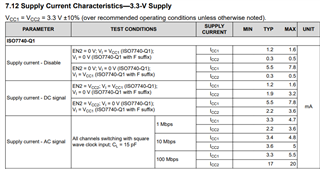# ISO7740-Q1: calculating worst case power draw

Part Number: ISO7740-Q1

Hi team,

I am trying to calculate the power draw in the worst case for the ISO7740FQ1 isolator IC. For static DC signals the power draw is assumed constant, right? Also assuming all channels (4 inputs) are high would the total current consumption be 4x ICC1 or just ICC1? Specifically speaking to Supply current – DC signal (Vi = VCC1, F suffix), so would be the worst case current be 7.8mA or 31.2mA?Errol

• Hi Errol,

Thank you for posting to E2E! For DC conditions, the worst-case current consumption is when the isolator inputs are in the devices' non-failsafe state. As you mentioned, this is when input signals are HIGH for "F"-suffix devices.

Since supply current parameters, Icc1 and Icc2, quantify the total current into each side of the isolator, it is not necessary to multiply them by the number of channels or input signals, and the "max" values for these specs already represent the worst-case. Below is an example calculation of the possible max power dissipation for Vcc1 = Vcc2 = 3.3V and V_IN = HIGH on an F-suffix device using the table you shared above:

max power dissipation = P_side1 + P_side2 = (3.3V x 7.8mA) + (3.3V x 3.6mA) =  37.62mW

Another example calculation can be found in the E2E thread linked below:If input signals are constant, the supply current values for DC signals should be used as in the calculation above, but if the signals toggle or oscillate, please use the AC signal parameters that match the frequency.